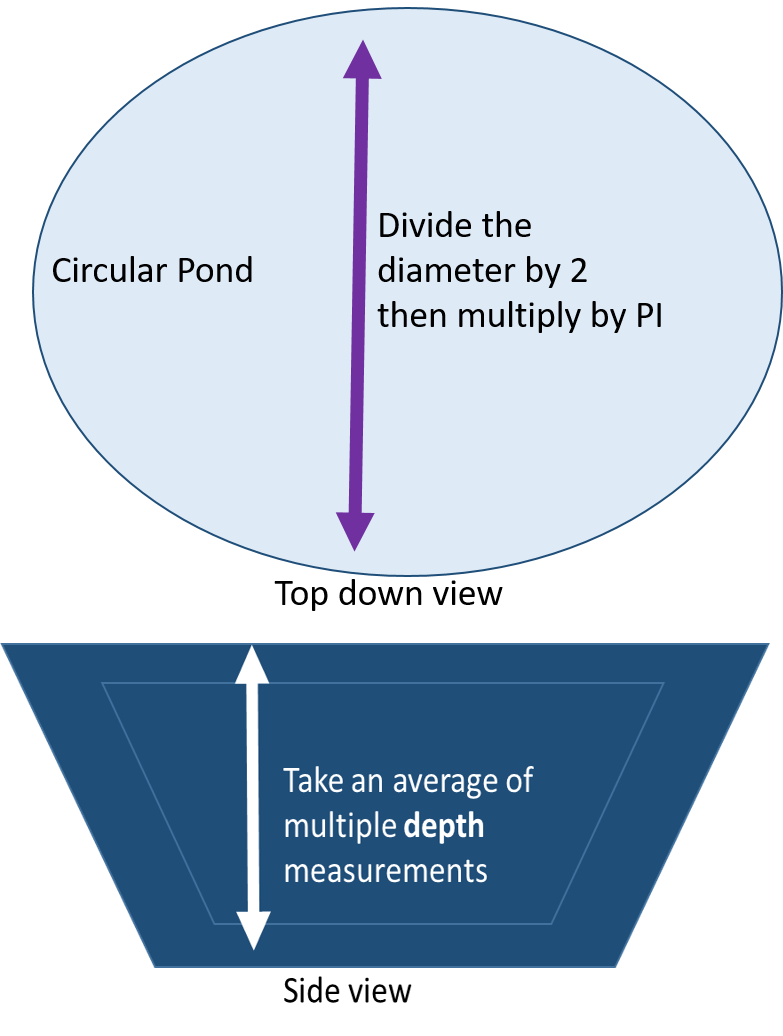# How much water is in my pond?

Many times, we are required to know the water volume of our pond for various applications. This includes size of fountains, fish carrying capacity, water treatments, and more.

Proper pond maintenance often requires knowing the volume of water that is in the pond. All package directions for any pond treatment, whether it is dye for controlling algae, chemicals to maintain pH balance, herbicides for managing weeds, determining the size of aerator to purchase, or determining how many fish you can stock, requires knowing the volume of water your pond contains. This information is critical for safe treatment of the water to ensure environmental safety and protect the health of the pond environment for the animals living there.

Determining this number is easy if your pond is one that you built yourself since that information may still be at hand. Unfortunately, or fortunately if you love math, you may have purchased your property with an existing pond where that information may not have been provided. Then you will need to calculate it yourself. It is not too difficult to do, but you will have to do some math. It is challenging to get exact measurements because it is hard to measure every part of most waterbodies. Taking an average gets you close enough for most applications and mixtures needed.

To start, first measure your pond across several places and then average the measurements based on the average shape of the body of water. Do this for the width and the length of your pond. These measurements will help you obtain the average surface area of the pond. Next, you will need to determine the average depth of your pond.

To establish the average depth of your pond you will need to take depth measurements along two perpendicular transects. Measure the depth in several places based on size and then average those numbers. Measure at least four different places in each direction; taking more measurements if the pond is large. Marking a rope with one-foot increments and adding a weight to the end is a helpful tool for depth measurements. Making more depth measurements will improve the accuracy of your final average value.Next use following formulas to calculate the size of your pond:

Square/ Rectangular (Gallons):

Assume the pond averages are 8 feet by 4 feet and averages 2 feet deep.

Multiply average length (feet) x average width (feet) x average water depth (feet) x 7.48 gallons per cubic foot.

Example: 8′ x 4′ x 2′ x 7.48 gallons = 479 gallons (rounded)Round (Gallons):

Assume the diameter is 10 feet and the average depth is 4 feet.

Divide the diameter (in feet) by 2 to get the radius. (Radius = ½ Diameter).

Then multiply the radius x itself x 3.14 (Pi) x the average water depth (feet) x 7.48 gallons per cubic foot. (The math here may be less intimidating if broken into smaller steps as in the example below.)

Example: 10 feet/2 feet =5 feet

(5 feet x 5 feet) x 3.14 = 78.5 square

(78.5 square feet x 4 feet) x 7.48 gallons per cubic foot =2350 gallons (rounded)Acres

There are 43,560 square feet in one acre of water that is1 foot deep. It is equal to 325,851 gallons. For a square pond, one acre is approximately 208 feet x 208 feet. It is important to note here that because of the increased size we are carrying our acre size calculations to three decimal points for more accurate results.

Square/Rectangular (Acres):

Assume the pond is 80 feet by 140 feet and is an average of 6 feet deep.

Multiply length x width, then divide by 43,560 (square feet per acre).

Then multiply this by the average depth in feet (acres x depth).

Example: 80′ x 140′ =11,200 square feet

11,200 square feet / 43,560 square feet per acre = .2571 acre (approximately ¼ of an acre)

.2571 acres x 6 feet = 1.543 acre-feet.

1.543 acre feet x 325,851 gallons/acre-foot = 502,788 gallons (rounded)

Round (Acres):

Assume the pond is 320 feet in diameter and averages 8 feet deep.

Divide the diameter (in feet) by 2 to get the radius. (Radius = ½ Diameter).

Then multiply the radius x itself x 3.14 (Pi) then divide by 43,560 (square feet per acre).

Then multiply this the average water depth (feet) x 325,851 gallons/acre foot.

Then multiply this by the foot depth, acres x depth.

Example: 320 feet/2 = 160 feet

160 feet x 160 feet x 3.14 = 80,384 square feet

80,384 square feet /43,560 square feet per acre =1.845 acres

1.845 acre x 8 feet x 325,851 gallons per acre/foot = 4,810,506 gallons

### Resources

Penn State Pond Measurements: Area, Volume and Residence Time

Cornell Calculating Water Volume in Ponds

An example of available online volume calculator applications and is installable on your smart device or Windows 10.

For more information about the Michigan Septic System Education, contact Beth Clawson, MSU Extension Educator. To learn more about this and other water quality programs, contact Michigan State University Extension  Natural Resources educators who are working across Michigan to provide natural resources and water quality educational programming and assistance. You can contact an educator through MSU Extension’s “Find an Expert” search tool using the keywords “Natural Resources” or “Water Quality.”